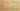# ThisCanBeNull

Misconception:

The special variable `this` can have the value `null`.Incorrect

this can be null

Correct

this is never null

## CorrectionHere is what's right.

The `this` variable (a special local variable) never is `null`. The variable exists during activations of instance methods, and it always points to the instance on which the method operates. That instance always exists, thus the `this` variable never is `null`.

Note that a call like `Object o = null; o.m();` would not succeed; it would throw a `NullPointerException`, and thus it defintely would not lead to a situation where `this` is `null`.

## OriginWhere could this misconception come from?

It is possible that this misconception originates in learning about recursive traversals over recursive structures. Students may see `null` as an object, and they may include checks for such a “base case” (`if (this == null)`) in implementations of methods of classes implementing recursive structures.

## SymptomsHow do you know your students might have this misconception?

The following question from a paper-based exam lead to a considerable number of student submissions exhibiting this misconception:

``````// The following class represents a recursive tree data structure.
// All your methods must be implemented using recursion.
public class Node {
private final Node left;
private final Node right;
private final int value;

public Node(Node left, Node right, int value) {
this.left = left;
this.right = right;
this.value = value;
}

/** Return the total number of nodes in this tree. */
public int count() {
// this body represents an implementation
// similar to those produced by students
if (this == null) return 0;
return left.count() + right.count() + 1;
}
}``````

The following code was produced by a student as part of a linked list implementation (after seeing a different implementation using a sentinel/null object, and having to produce an implementation that does not use a null object to terminate the list):

``````public class IntList {

private int value;
private IntList tail;

public int length() {
if (this==null) {
return 0;
} else if (this.tail == null) {
return 1;
} else {
this.tail.length();
}
}
}``````

Java